Want to share your content on R-bloggers? click here if you have a blog, or here if you don't.# Searching for Speed

In my previous post, I described the method I use for compiling Fortran (or C) into an R package using the `.Call` interface. This post will compare the speed of various implementations of the layer loss cost function.

# The Function

Often, insurance or reinsurance is bought in stratified horizontal layers. For example, an auto policy with a \$300,000 limit may have its middle third insured. This means that the primary insurer would pay up to the first \$100,000 of loss, a reinsurer would pay the next \$100,000, and the primary insurer would pay the last \$100,000. In actuarial terms, we say that the reinsurer is covering \$100K excess of \$100K. The reinsured losses attach at \$100K and are limited to a total of \$100K paid loss. Ifis the random variable representing the loss,is the limit of the policy, and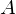the attachment, then the layer loss cost, LLC is:In R, this is extremely easy to write:

`LLC_r <- function(x, l, a) sum(pmax(0, pmin(x - a, l)))`

# The Question

The two main languages which comprise the compiled code in R are C/C++ and Fortran. I’m not sure why, but I have a soft spot in my heart for Fortran, specifically the free-form, actually readable, versions of Fortran from F90 and on. For the packages I maintain on CRAN, I’ve found that, more often than not, Fortran will outperform C++ by a small margin—although not always. This was opportunity to directly compare the performance of C++ and Fortran.

## Parallelization

### Basics

Speed improvements often come from explicit parallelization of the compiled code. In parallel computing, vectorization means using the multiple SIMD lanes in modern chips (SSE/AVX/AVX2). The loop is only performed in one thread but it can be chunked inside the CPU. Consider a one-lane highway approaching a toll plaza; the speed limit remains the same but just at the tollbooth four cars can be processed at the same time. Parallelization means taking advantage of multiple cores. This is akin to splitting the one lane into several lanes, each one with its own speed limit and approach to its own toll-plaza. Sometimes single-thread vectorization is called “fine-grain parallelism” and multi-thread parallelism is called “coarse-grain parallelism”. Both can be combined on today’s modern SIMD CPUs with multiple cores.

In theory, either of these should be faster than single-thread serial calculation. In practice, however, there is a certain amount of overhead involved in setting up either kind of parallelism. Therefore, for small loads, it is not uncommon to find the serial versions to be the fastest. Even for larger, or huge, loads, it is not always the case that the most parallelism is worth the most overhead. In each language tested, the code will test coarse-grained, fine-grained, and combined parallelism.

### R-specific hurdle

The standard way to parallelize C/C++/Fortran is by using OpenMP. However, R’s mechanics officially don’t allow OpenMP to be used by multiple languages in the same package. While it may be forced, the forcing violates R norms and relies on some default linker flags being the same across languages. Even now, December 2018, the Fortran mechanics for OpenMP are changing. So to test the languages, I wrote two small packages—one exclusively C++ using Rcpp and one using the C/Fortran interface. In the end, I did violate those norms to show some pure C calls which were put into the Fortran package. While this will work for now and for this post, it is not good practice and should be avoided.

# Approaches

## R

In base R, the pure translation would be the nested use of `pmin` and `pmax`. There is one variant; the documentation for the two functions also describes a `.int` version for both which are “faster internal versions only used when all arguments are atomic vectors and there are no classes.” So for R, there are two options to be checked:

```LLC_r1 <- function(x, l, a) sum(pmax(0, pmin(x - a, l)))
LLC_r2 <- function(x, l, a) sum(pmax.int(0, pmin.int(x - a, l)))```

## C++/Rcpp

### Serial Loop

Unlike R, the easiest way to calculate this function in C++ is to use a for loop. There may be more elegant ways using STL, but those are more difficult to implement (and the LLC function isn’t unary anyway). In R, loops are deprecated in favor of vectorized functions and the `apply` family. The reason is that the looping is handled by the underlying C/C++/Fortran and not at the R level. Here we are living in the C++ basement, so well-formed loops are both good and fast. The archetypal loop implementation for the layer loss cost function in Rcpp would be:

```// [[Rcpp::export]]
double LLC_cpp(NumericVector X, double L, double A) {
int n = X.size();
double LLC = 0.0;
for (int i = 0; i < n; ++i) {
LLC += fmax(0.0, fmin(X[i] - A, L));
}
return(LLC);
}```

Not only is this implementation fast, a sufficiently good and aggressive compiler can also attempt autovectorization for even more speed (`-O3` will usually attempt this). However, explicit vectorization and parallelization for C/C++ and Fortran will be applied using OpenMP. There are other ways to impose parallelism, like the Intel TBB framework behind RcppParallel. In that lies a possible addendum or two to this post.

### Parallel Loops

Three additional flavors of the C++ code were tested:

• Only using SIMD vectorization: `LLC_cpps`
• Only using explicit parallization: `LLC_cppl`
• Using both: `LLC_cppls`

The entire `.cpp` file is posted below to show the includes and other Rcpp necessities which weren’t covered in the first post.

```#include
#include
#include
using namespace Rcpp;

//[[Rcpp::plugins(openmp)]]

// [[Rcpp::export]]
double LLC_cpp(NumericVector X, double L, double A) {
int n = X.size();
double LLC = 0.0;
for (int i = 0; i < n; ++i) {
LLC += fmax(0.0, fmin(X[i] - A, L));
}
return(LLC);
}

// [[Rcpp::export]]
double LLC_cpps(NumericVector X, double L, double A) {
int n = X.size();
double LLC = 0.0;
#pragma omp simd reduction(+: LLC)
for (int i = 0; i < n; ++i) {
LLC += fmax(0.0, fmin(X[i] - A, L));
}
return(LLC);
}

// [[Rcpp::export]]
double LLC_cppl(NumericVector X, double L, double A) {
int n = X.size();
double LLC = 0.0;
#pragma omp parallel for schedule(static), reduction(+:LLC)
for (int i = 0; i < n; ++i) {
LLC += fmax(0.0, fmin(X[i] - A, L));
}
return(LLC);
}

// [[Rcpp::export]]
double LLC_cppls(NumericVector X, double L, double A) {
int n = X.size();
double LLC = 0.0;
#pragma omp parallel for simd schedule(static), reduction(+:LLC)
for (int i = 0; i < n; ++i) {
LLC += fmax(0.0, fmin(X[i] - A, L));
}
return(LLC);
}```

The `reduction` code word lets the compiler know which variable is the sum accumulator to which the separate threads or vectors need to return their work. The `schedule` keyword tells the compiler how to split up the work into separate threads. The `static` option causes the work to be split into evenly-sized chunks. In OpenMP 4.5, there is a special scheduling option for loops with SIMD, but the current Rtools for Windows is based on GCC 4.9.3, which is limited to OpenMP 4.0.

Using OpenMP requires some special handling in the Makevars[.win] file. The entire package including the C++ code, Makevars, and other goodies can be found at: https://github.com/aadler/CppLangComp

## Fortran

### Direct

Unlike C/C++, arrays are first-class citizens in modern Fortran (F90+). Therefore, the function can be written without explicit looping. Fortran knows `x` is an array, and will behave accordingly. The function below is named `llc_fd` for “Fortran – Direct”:

```subroutine llc_fd(x, n, l, a, llc) bind(C, name = "llc_fd_")

real(kind = c_double), intent(in)               :: l, a
integer(kind = c_int), intent(in), value        :: n
real(kind = c_double), intent(in), dimension(n) :: x
real(kind = c_double), intent(out)              :: llc

llc = sum(max(0.0_c_double, min(x - a, l)))

end subroutine llc_f1```

Another element (pun intended) of Fortran is the concept of elemental functions. These are functions which take scalar inputs, return scalar outputs, and have no side effects. For example, the Fortran intrinsic `log` function takes a scalar input, returns the log of that value as output, and does nothing else. The benefit of creating an elemental functions is that the Fortran compiler recognizes the potential for automatically vectorizing/parallelizing the function when applied to an array. So a second direct approach would be to make the calculation of the LLC an elemental function and apply that to the vector. The function below is named `llc_fe` for “Fortran – Elemental”:

```elemental function llce(x, l, a) result(y)

real(kind = c_double), intent(in)               :: x, l, a
real(kind = c_double)                           :: y

y = max(0.0_c_double, min(x - a, l))

end function llce

subroutine llc_fe(x, n, l, a, llc) bind(C, name = "llc_fe_")

real(kind = c_double), intent(in)               :: l, a
integer(kind = c_int), intent(in), value        :: n
real(kind = c_double), intent(in), dimension(n) :: x
real(kind = c_double), intent(out)              :: llc

llc = sum(llce(x, l, a))

end subroutine llc_fe```

In Fortran, `max` and `min` are themselves elemental functions, so the “direct” implementation is actually elemental as well. Thus, it will probably be a tick faster, as it doesn’t need the intermediary `llce` function.

### Serial Loop

Loops in Fortran tend to be very fast, especially when using `-O3`, as the compilers unroll/autovectorize pretty decently. The function below is named `llc_f` as it’s the basic Fortran implementation most comparable to its Rcpp/C++ and C counterparts:

```subroutine llc_f(x, n, l, a, llc) bind(C, name = "llc_f_")

real(kind = c_double), intent(in)               :: l, a
integer(kind = c_int), intent(in), value        :: n
real(kind = c_double), intent(in), dimension(n) :: x
real(kind = c_double), intent(out)              :: llc
integer                                         :: i

llc = 0.0_c_double
do i = 1, n
llc = llc + max(0.0_c_double, min(x(i) - a, l))
end do

end subroutine llc_f```

### Parallel Loops

Similar to the Rcpp/C++ versions, there are three parallelized loop versions: SIMD only, parallelization only, and both. The parallel functions follow similar naming to the Rcpp versions above. To see the entire `.f95` file, see the source file on github:

```subroutine llc_fs(x, n, l, a, llc) bind(C, name = "llc_fs_")

real(kind = c_double), intent(in)               :: l, a
integer(kind = c_int), intent(in), value        :: n
real(kind = c_double), intent(in), dimension(n) :: x
real(kind = c_double), intent(out)              :: llc
integer                                         :: i

llc = 0.0_c_double
!\$omp do simd reduction(+:llc)
do i = 1, n
llc = llc + max(0.0_c_double, min(x(i) - a, l))
end do
!\$omp end do simd

end subroutine llc_fs

subroutine llc_fl(x, n, l, a, llc) bind(C, name = "llc_fl_")

real(kind = c_double), intent(in)               :: l, a
integer(kind = c_int), intent(in), value        :: n
real(kind = c_double), intent(in), dimension(n) :: x
real(kind = c_double), intent(out)              :: llc
integer                                         :: i

llc = 0.0_c_double
!\$omp parallel do reduction(+:llc), schedule(static), private(i)
do i = 1, n
llc = llc + max(0.0_c_double, min(x(i) - a, l))
end do
!\$omp end parallel do

end subroutine llc_fl

subroutine llc_fls(x, n, l, a, llc) bind(C, name = "llc_fls_")

real(kind = c_double), intent(in)               :: l, a
integer(kind = c_int), intent(in), value        :: n
real(kind = c_double), intent(in), dimension(n) :: x
real(kind = c_double), intent(out)              :: llc
integer                                         :: i

llc = 0.0_c_double
!\$omp parallel do simd reduction(+:llc), schedule(static)
do i = 1, n
llc = llc + max(0.0_c_double, min(x(i) - a, l))
end do
!\$omp end parallel do simd

end subroutine llc_fls```

## C

Now that we know how to fold in compiled code, we can write pure(ish) C functions and compare them to both the Fortran and Rcpp/C++. The same four versions as the Rcpp functions can be written directly in C and are named `c_llc_c, c_llc_cs, c_llc_cl, c_llc_cls` describing their parallelization. Per Hadley Wickham’s advice, it is faster to move the singleton SEXPs into local constant variables, and to declare a pointer to the vector SEXP, than it is to call them directly inside the loop. To see the entire `.c` file with the includes, the Fortran interfaces, and the R registration functions, please see the source file on github:

```extern SEXP c_llc_c(SEXP x, SEXP l, SEXP a){
const int n = LENGTH(x);
const double ll = asReal(l);
const double aa = asReal(a);
double *px = REAL(x);
double llc = 0.0;
for (int i = 0; i < n; ++i) {
llc += fmax(0.0, fmin(px[i] - aa, ll));
}
SEXP ret = PROTECT(allocVector(REALSXP, 1));
REAL(ret) = llc;
UNPROTECT(1);
return(ret);
}

extern SEXP c_llc_cs(SEXP x, SEXP l, SEXP a){
const int n = LENGTH(x);
const double ll = asReal(l);
const double aa = asReal(a);
double *px = REAL(x);
double llc = 0.0;
#pragma omp simd reduction(+:llc)
for (int i = 0; i < n; ++i) {
llc += fmax(0.0, fmin(px[i] - aa, ll));
}
SEXP ret = PROTECT(allocVector(REALSXP, 1));
REAL(ret) = llc;
UNPROTECT(1);
return(ret);
}

extern SEXP c_llc_cl(SEXP x, SEXP l, SEXP a){
const int n = LENGTH(x);
const double ll = asReal(l);
const double aa = asReal(a);
double *px = REAL(x);
double llc = 0.0;
#pragma omp parallel for schedule(static), reduction(+:llc)
for (int i = 0; i < n; ++i) {
llc += fmax(0.0, fmin(px[i] - aa, ll));
}
SEXP ret = PROTECT(allocVector(REALSXP, 1));
REAL(ret) = llc;
UNPROTECT(1);
return(ret);
}

extern SEXP c_llc_cls(SEXP x, SEXP l, SEXP a){
const int n = LENGTH(x);
const double ll = asReal(l);
const double aa = asReal(a);
double *px = REAL(x);
double llc = 0.0;
#pragma omp parallel for simd schedule(static), reduction(+:llc)
for (int i = 0; i < n; ++i) {
llc += fmax(0.0, fmin(px[i] - aa, ll));
}
SEXP ret = PROTECT(allocVector(REALSXP, 1));
REAL(ret) = llc;
UNPROTECT(1);
return(ret);
}```

## Necessary Evils

The `.R` file is straightforward, especially after the previous post. The necessary evil is the `Makevars` in which I violated my own warning about having OpenMP called by two languages in the same package. The entire package, which is not CRAN-worthy, can be found on github.

## Tests

There are sixteen implementations of the LLC function between the two packages. These were tested with 1000 replications of calculating the layer loss cost for a \$2M excess of \$1M layer for sequences of 1, 10, 100, 1000, 10K, 100K, and 1M generated losses. Timing was done using the microbenchmark package.

The timing code can be found as Timings.R in the `inst/Timings` directory of the package. In that script, the last commented-out call will create a nice table of all the timings. As that would have overwhelmed this article, it isn’t posted here.

# Results

## Synopsis

For the layer loss cost function, Fortran consistently outperforms its equivalent C and C++ routines. For vectors up to around length 1000, the Fortran serial loop, direct, and elemental versions are approximately the same. Between 1000 and almost 100K, the Fortran serial loop is the fastest. Shortly before 100K, the overhead of the parallelization is overtaken by the speed of using multiple cores. The parallelization overhead is significant enough that even base R outperforms the compiled code up to almost 10K elements. Also, using gcc/gfortran 4.9.3, there is no real benefit using SIMD. Serial SIMD is equivalent to serial loops and parallel SIMD is equivalent to parallel loops. Lastly, the `.int` version of `pmin` and `pmax` is faster up to about vectors of length 10K, after which is about the same as the regular version. Select the graph below for a larger version.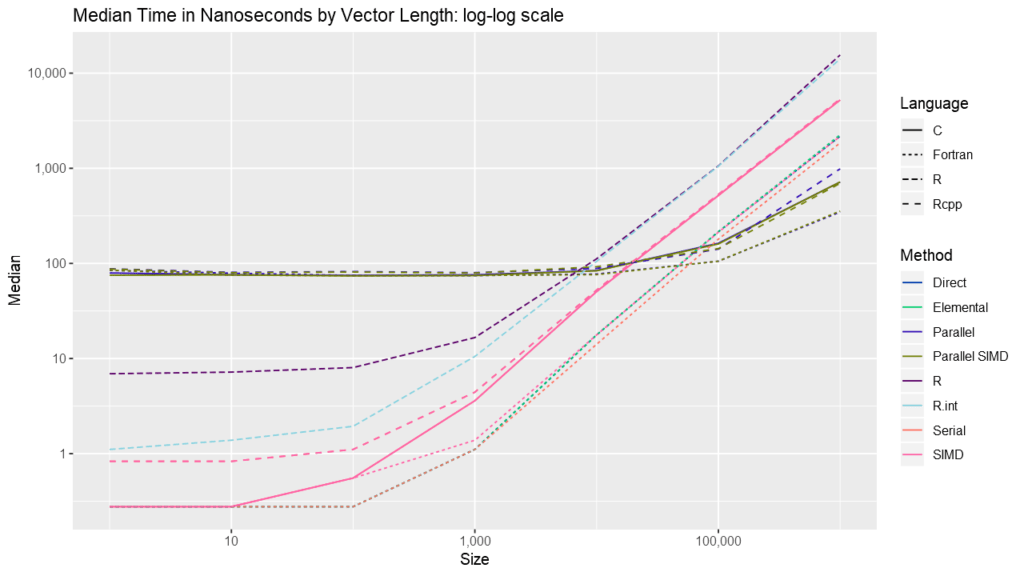In closing, significant speed can be achieved in R by using compiled code. For this function, Fortran outperformed C and Rcpp, but that is not always the case. Hopefully, after these two posts, it will be easier for the interested reader to experiment, especially when needing to squeeze out every drop of speed.

## Equivalently-sized Vector Comparisons

Below are a series of box and ridge plots comparing the distribution of the 1000 results across language and method. There are no ridge plots for lengths less than 1000 as ggridges returned an error for those. All graphs can be selected for a larger view.

### Box Plots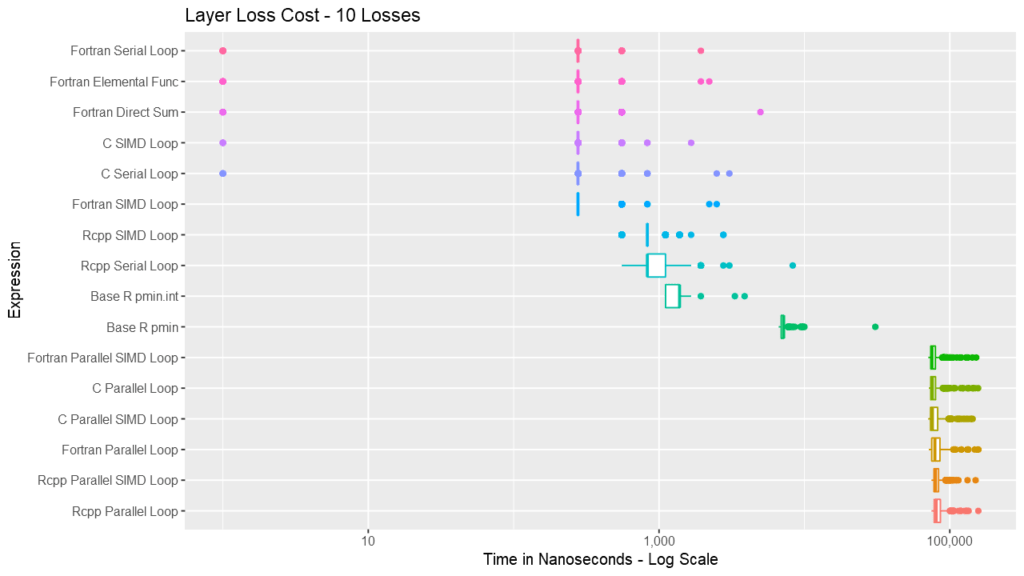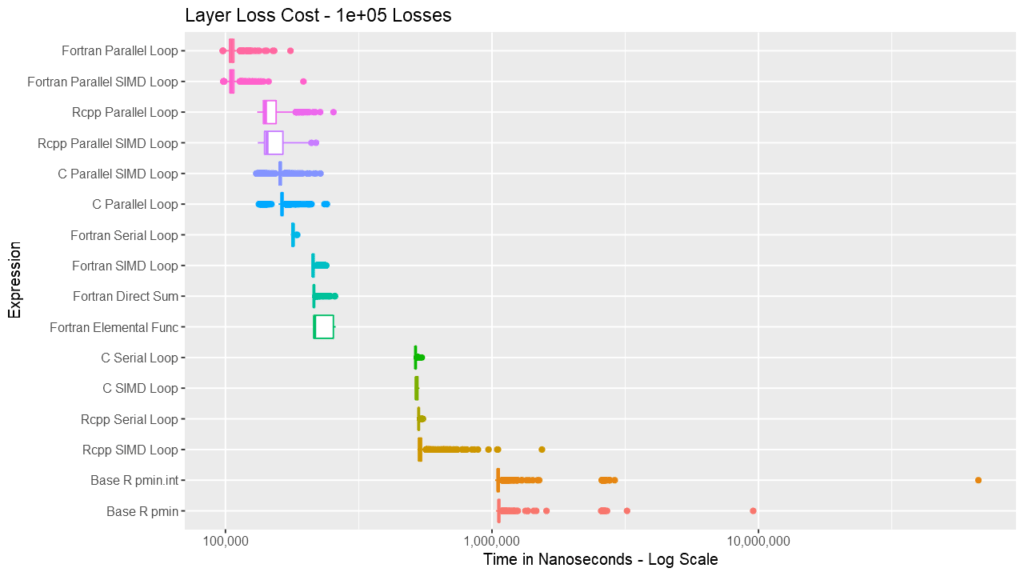### Ridge Plots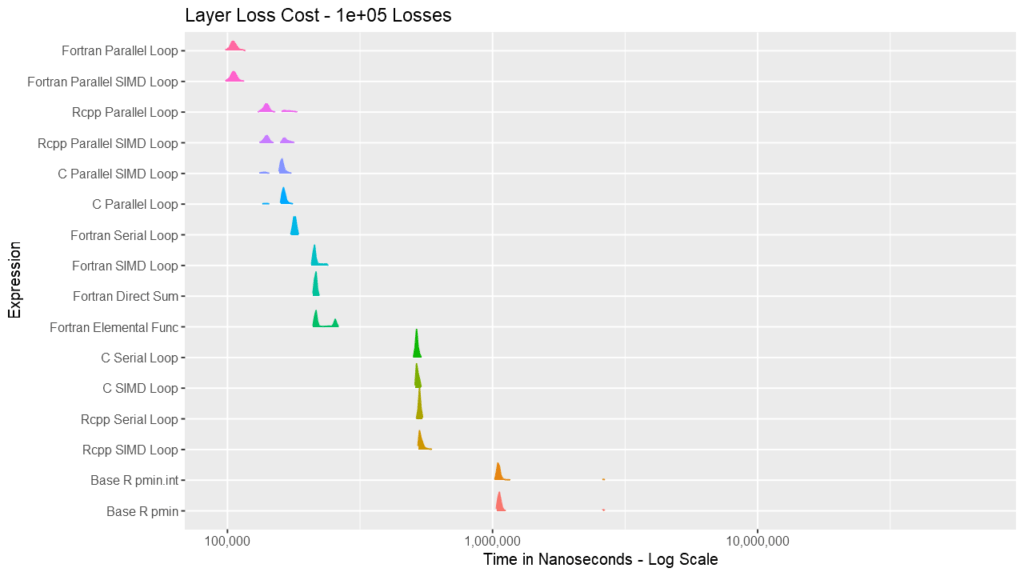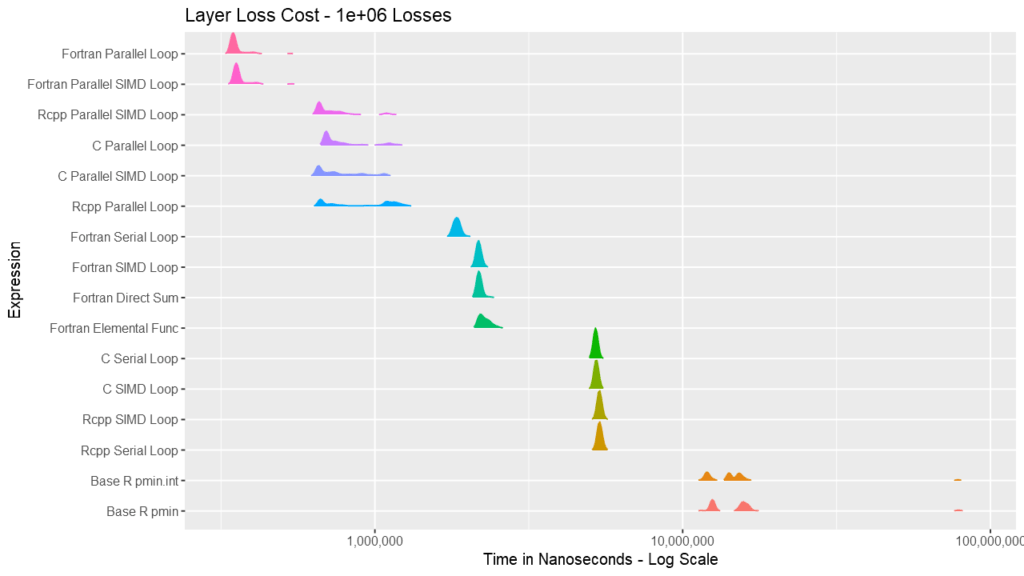# SessionInfo

These timings were performed on an i7-8700K overclocked to 5.03Ghz, ASUS ROG MAXIMUS X HERO Z370 motherboard, 32Gb RAM, 960 Pro NVME hard drive, NVIDIA GTX 1080Ti.

```R version 3.5.0 Patched (2018-05-16 r74732)
Platform: x86_64-w64-mingw32/x64 (64-bit)
Running under: Windows >= 8 x64 (build 9200)

Matrix products: default

locale:
 LC_COLLATE=English_United States.1252  LC_CTYPE=English_United States.1252    LC_MONETARY=English_United States.1252
 LC_NUMERIC=C                           LC_TIME=English_United States.1252

attached base packages:
 stats     graphics  grDevices utils     datasets  methods   base

other attached packages:
 data.table_1.11.8    ggridges_0.5.1       scales_1.0.0         ggplot2_3.1.0        microbenchmark_1.4-6 CppLangComp_0.0.1    RFortLangComp_0.0.1

loaded via a namespace (and not attached):
 Rcpp_1.0.0       rstudioapi_0.8   bindr_0.1.1      magrittr_1.5     tidyselect_0.2.5 munsell_0.5.0    colorspace_1.3-2 R6_2.3.0         rlang_0.3.0.1
 plyr_1.8.4       dplyr_0.7.8      tools_3.5.0      grid_3.5.0       gtable_0.2.0     withr_2.1.2      yaml_2.2.0       lazyeval_0.2.1   assertthat_0.2.0
 tibble_1.4.2     crayon_1.3.4     bindrcpp_0.2.2   purrr_0.2.5      glue_1.3.0       compiler_3.5.0   pillar_1.3.0     pkgconfig_2.0.2```

The post The Need for Speed Part 2: C++ vs. Fortran vs. C appeared first on Strange Attractors.